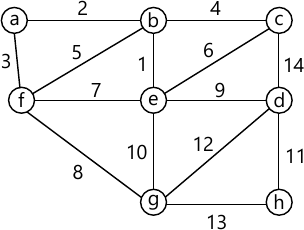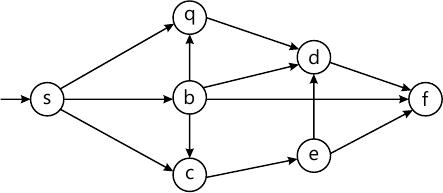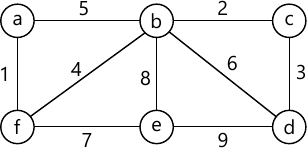MORE IN Data Structures Algorithm
SPPU Computer Engineering (Semester 3)
Data Structures Algorithm
December 2015
Total marks: --
Total time: --
INSTRUCTIONS
(1) Assume appropriate data and state your reasons
(2) Marks are given to the right of every question
(3) Draw neat diagrams wherever necessary

Solve any one question from Q1 and Q2
1 (a) Write the frequency count of the following code and derive the time complexity.
For (i=n-1; i>0; i--)
For (j=0; j<1; j++)
If (a[i]     {
Temp=a[i];
a[i]=a[i+1];
a[i+1]=temp;
}
4 M
1 (b) Prove the following:
i) If f(n)=2n2+2 then f(n)ϵ O(n2)
ii) if f(n)=5n3+2n2+3 then f(n) ϵ O(n3).
4 M
1 (c) Prove that if height of a full or couple binary tree is 'h' then number of nodes in the tree equal to 2h+1-1.
4 M

2 (a) Prove that a full binary tree having n nodes, the height is O(log2n).
3 M
2 (b) Evaluate the following postfix expression using stack. Show all steps.
3,2,4,*,+,5,9,3,/,+,*.
3 M
2 (c) Define Big O, Ω and θ.
3 M
2 (d) Show analysis of quick sort in worst and best case.
3 M

Solve any one question from Q3 and Q4
3 (a) Find the minimum spanning tree for the following graph using Kruskal's Algorithms.4 M
3 (b) Find the topological ordering of the following graph:4 M
3 (c) Construct the AVL tree for the following data:
5, 4, 7, 1, 3, 2, 15, 20, 10, 12.
4 M

4 (a) Insert the following data in the has table of size 10, using linear probing with chaining with replacement.
Here h(x)=x%10
21, 35, 31, 37, 32, 33, 48.
4 M
4 (b) Write 'C' code for the following functions w.r.t. AVL Tree:
i) LR Rotation
ii) RL Rotation.
4 M
4 (c) Find the minimum spanning tree for the following graph using Prim's Algorithms.4 M

Solve any one question from Q5 and Q6
5 (a) Construct B tree of order 5 for the following data:
4, 8, 10, 5, 3, 9, 2, 15, 20, 80.
5 M
5 (b) Sort the following data in ascending order using heap sort:
10, 5, 3, 8, 9, 4, 2.
4 M
5 (c) Explain various operations on sequential files.
4 M

6 (a) Construct B+ Tree of the order 5 for the following data:
5, 4, 6, 2, 1, 7, 1, 8, 9, 3, 10.
5 M
6 (b) Explain with example different methods of heap creation, also explain which method is better and why?
4 M
6 (c) Write short notes on:
i) Sequential files
ii) Random access files.
4 M

Solve any one question from Q7 and Q8
7 (a) Computer the prefix sum for the following list using list ranking:
5, 3, -2, 7, 6.
4 M
7 (b) Explain pointer jumping techniques.
3 M
7 (c) Write a note on odd even merge sort.
3 M
7 (d) Find the largest number in the following list using parallel algorithmic technique:
5, 3, 7, 8, 2.
3 M

8 (a) Explain different parallel algorithmic techniques with examples.
6 M
8 (b) Explain list ranking problem using pointer jumping techniques. Compute prefix sum of (8, 2, -1, 5) using binary tree techniques.
4 M
8 (c) Compute the sum of the following numbers using complete binary tree technique:
5, 4, 3, 2.
3 M

More question papers from Data Structures Algorithm